## LetsPlayMaths.Com

WELCOME TO THE WORLD OF MATHEMATICS

Step 1. Write both the numbers in tabular format.

Step 2. Add the ones together and write the result in one’s column.

Step 3. Add the tens together and write the result in ten’s column.

Step 4. Add the hundred together and write the result in hundred’s column.

Let’s have a look at some examples.

Example 1. Add 54 and 32.

Solution.
Step 1. We must write 54 and 32 in tabular format as shown below.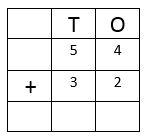Step 2. Add the digits present in ones column and write the result in ones column.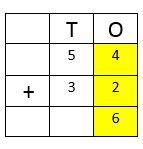Step 3. Add the digits present in tens column and write the result in tens column.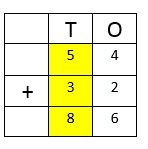So, the result is 86.

Examples 2. Add 256 and 623.

Solution. Write 256 and 623 in tabular format.Add the digits present in ones column and write the result in ones column.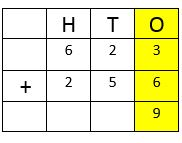Add the digits present in tens column and write the result in tens column.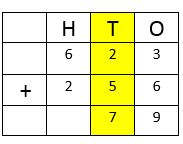Add the digits present in hundreds column and write the result in hundreds column.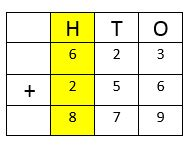So, the result is 879.

Example 3. Add 231, 145 and 12

Solution. Write 231, 145 and 12 in tabular format.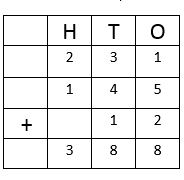Example 1. Add 65 and 26.

Solution.Write 65 and 26 in tabular format as shown below.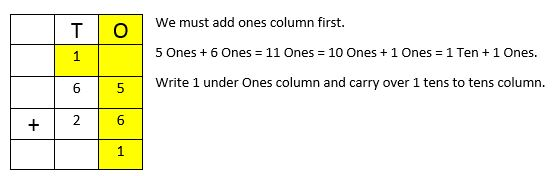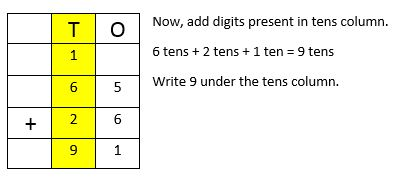Example 2. Add 357 and 245.

Solution.Write 357 and 265 in tabular format as shown below.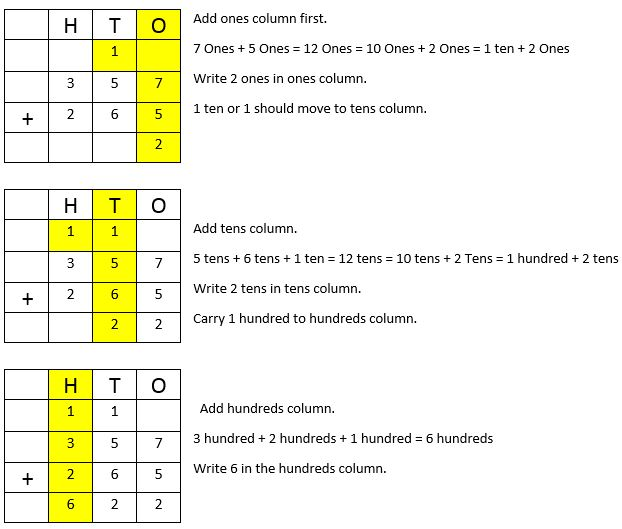Example 3. Add 345, 243 and 134.

Solution. Write 345, 253 and 134 in tabular format.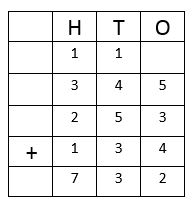In our day to day life we use concept of addition in many ways, few examples are shown below.

Example 1. Bob has 45 pencils with him. His father gave him 27 pencils more, then how many pencils he has in total?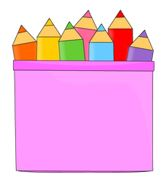Solution.Bob has 45 pencils
Bob’s father gave him 27 more pencils

Total number of pencils Bob has = 45 pencils + 27 pencils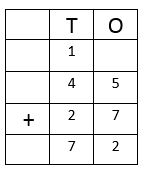So, Bob has 72 pencils with him.

Example 2. In a school library there were 267 books. School bought 127 books more, then what is the total number books now?Solution.School library has 267 books

School bought 127 more books

Total number of books = 267 books + 127 books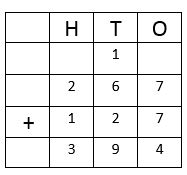So, the school has 394 books in total.